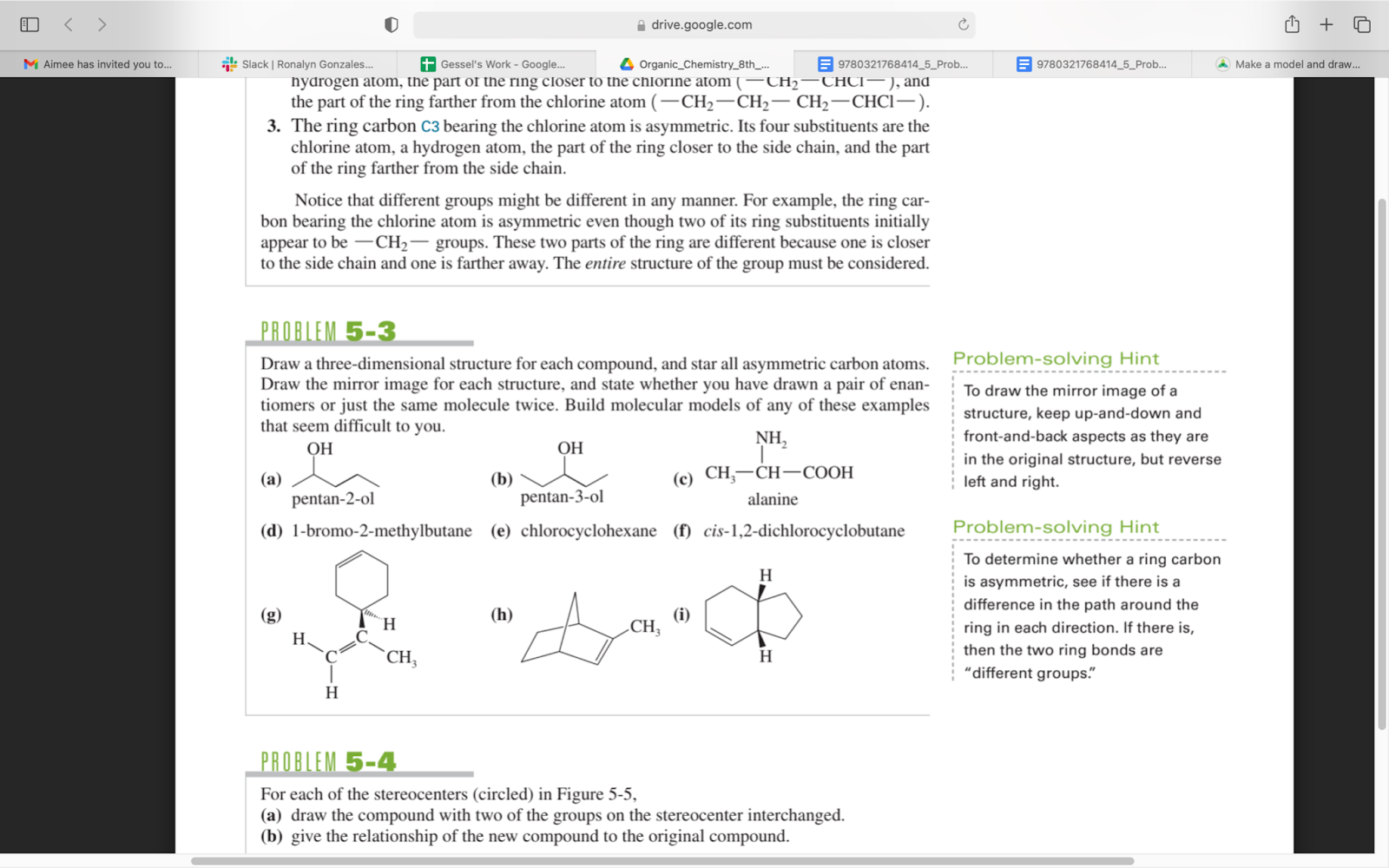×
Get Full Access to Organic Chemistry - 8 Edition - Chapter 5 - Problem 3p
Get Full Access to Organic Chemistry - 8 Edition - Chapter 5 - Problem 3p

×

# Draw a three-dimensional structure for each compound, andISBN: 9780321768414 33

## Solution for problem 3P Chapter 5

Organic Chemistry | 8th Edition

• Textbook Solutions
• 2901 Step-by-step solutions solved by professors and subject experts
• Get 24/7 help from StudySoup virtual teaching assistantsOrganic Chemistry | 8th Edition

4 5 1 281 Reviews
12
2
Problem 3P

Draw a three-dimensional structure for each compound, and star all asymmetric carbon atoms. Draw the mirror image for each structure, and state whether you have drawn a pair of enantiomers or just the same molecule twice. Build molecular models of any of these examples that seem difficult to you.Equation Transcription:Text Transcription:

OH

OH

CH3-CH-NH3-COOH

CH3

CH3

Step-by-Step Solution:

Solution 3P

Step 1Step 2 of 17

Step 3 of 17

## Discover and learn what students are asking

Calculus: Early Transcendental Functions : Slope Fields and Eulers Method
?In Exercises 1-8, verify the solution of the differential equation. Solution D

Statistics: Informed Decisions Using Data : Discrete Probability Distributions
?Determine whether the distribution is a discrete probability distribution. If not, state why.

Unlock Textbook Solution